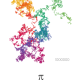# Union, Intersection, and DifferenceLevi

Really liking the breakdown of this.Sciencificity

Hi James, I think that `symmetric_difference()` only takes one argument since it does not make sense when you apply it to multiple arguments. This is due to the left to right nature of the operator. The in between sets used with `^` can introduce elements that appeared in previous sets but were discarded by the previous use of `^`. E.g using the example we used previously we would have the below:

``````a = {1,2,3,4}
b = {2,3,4,5}
c = {3,4,5,6}
d = {4,5,6,7}
print(a ^ b ^ c ^ d) # a ^ b -> {1,5} ^ c -> {1,3,4,6} ^ d -> {1,3,5,7}

{1, 3, 5, 7} # result ... but 3, 5 occur in multiple sets
``````

Also if we amend the example used in the video slightly (add a 2 in last set):

``````a = {1,2,3,4,5}
b = {10,2,3,4,50}
c = {1,2,50,100}
print(a ^ b ^ c)

{2, 100, 5, 10} # result
``````Kumaran Ramalingam

Hi James, It was really useful for me to breakdown things and learn

to join the conversation.

Lesson Completed!
Lesson Bookmarked
Request Failed :(